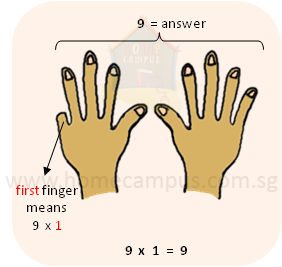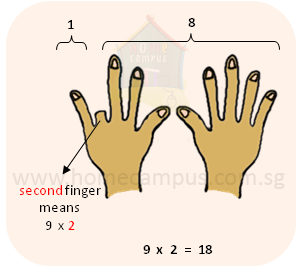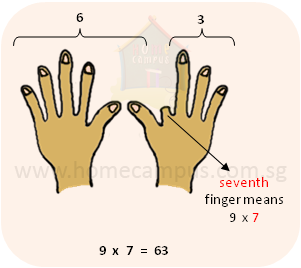## Tips to Remember Multiplication / Times Tables of 6, 7, 8 and 9

Times Table Chart and Calculator

#### Times table of 6

Method 1:

• For 6  ×  an even number (2, 4, 6 or 8 (NOT 10 and 12))

• The digit in the ones place will be the same as that even number.

• The digit in the tens place will be half of that even number.

So,

6  ×  2  =  12

6  ×  4  =  24

6  ×  6  =  36

6  ×  8  =  48

• For 6  ×  an odd number (3, 5, 7, 9 or 11)

• Go to the even number just before it and multiply it by 6.

So,

6  ×  3  =  (6  ×  2) + 6  =  12 + 6  =  18

6  ×  5  =  (6  ×  4) + 6  =  24 + 6  =  30

6  ×  7  =  (6  ×  6) + 6  =  36 + 6  =  42

6  ×  9  =  (6  ×  8) + 6  =  48 + 6  =  54

6  ×  11 = (6  ×  10) + 6  =  60 + 6 = 66

METHOD 2:

6 is the double of 3. So, first do 3  ×  and then double the result to get the answer.

Examples:

6  ×  4

• Step 1: 3  ×  4 = 12

• Step 2: 12 + 12 = 24

• So, 6  ×  4 = 24

6  ×  8

• Step 1: 3  ×  8 = 24

• Step 2: 24 + 24 = 48

• So, 6  ×  8 = 48

6  ×  12

• Step 1: 3  ×  12 = 36

• Step 2: 36 + 36 = 72

• So, 6  ×  12 = 72
6  ×  1  =  6

6  ×  2  =  12

6  ×  3  =  18

6  ×  4  =  24

6  ×  5  =  30

6  ×  6  =  36

6  ×  7  =  42

6  ×  8  =  48

6  ×  9  =  54

6  ×  10  =  60

6  ×  11  =  66

6  ×  12  =  72

#### Times table of 7

7× is slightly tricky but not that bad!! So, here we go...
• For 2 to 5, switch the numbers.

7 × 2   =   2 × 7   =   14

7 × 3   =   3 × 7   =   21

7 × 4   =   4 × 7   =   28

7 × 5   =   5 × 7   =   35

• After that, use 7 × 5 = 35  or  7 × 10 = 70 to get the rest.

7 × 6  =  35 + 7   =   42

7 × 7  =  35 + 7 + 7   =   49

7 × 8  =  35 + 7 + 7 + 7   =   56

7 × 9  =  70 − 7   =   63

7 × 10  =  70

7 × 11  =  70 + 7   =   77

7 × 12  =  70 + 7 + 7   =   84

7  ×  1  =  7

7  ×  2  =  14

7  ×  3  =  21

7  ×  4  =  28

7  ×  5  =  35

7  ×  6  =  42

7  ×  7  =  49

7  ×  8  =  56

7  ×  9  =  63

7  ×  10  =  70

7  ×  11  =  77

7  ×  12  =  84

#### Times table of 8

METHOD 1:
8 is the double of 4. So, first do 4× and then double the result to get the answer.

Examples:

8 × 3

• Step 1: 4 × 3  =  12

• Step 2: 12  +  12  =  24

• 8  ×  3  =  24

8 × 7
 Step 1: 4 × 7 = 28 Step 2: 28  +  28 = 56 So, 8  ×  7 = 56

8 × 12
 Step 1: 4 × 12 = 48 Step 2: 48  +  48 = 96 So, 8  ×  12 = 96

METHOD 2:
8  =  10  −  2
So,
8×  =  10×  −  2×

Examples:
8 × 3
 Step 1: 10 × 3 = 30 Step 2: 2 × 3 = 6 Step 3: 30 − 6 = 24 So, 8  ×  3 = 24

8 × 7
 Step 1: 10 × 7 = 70 Step 2: 2 × 7 = 14 Step 3: 70 − 14 = 56 So, 8  ×  7 = 56

8 × 12
 Step 1: 10 × 12 = 120 Step 2: 2 × 12 = 24 Step 3: 120 − 24 = 96 So, 8  ×  12 = 96
8  ×  1  =  8

8  ×  2  =  16

8  ×  3  =  24

8  ×  4  =  32

8  ×  5  =  40

8  ×  6  =  48

8  ×  7  =  56

8  ×  8  =  64

8  ×  9  =  72

8  ×  10  =  80

8  ×  11  =  88

8  ×  12  =  96

#### Times table of 9

METHOD 1:   Use the finger counting method.

Examples:
9 × 1
 Bend your first finger and count as shown.9 × 2
 Bend your second finger and count as shown below.9 × 7
 Bend your seventh finger and count as shown below.METHOD 2:   Do 10× the number minus the number.

Examples:
9 × 2  =  (10 × 2) − 2   =   20 − 2  = 18

9 × 7  =  (10 × 7) − 7   =   70 − 7  = 63

9 × 12 = (10 × 12) − 12 = 120 − 12 =108

9  ×  1  =  9

9  ×  2  =  18

9  ×  3  =  27

9  ×  4  =  36

9  ×  5  =  45

9  ×  6  =  54

9  ×  7  =  63

9  ×  8  =  72

9  ×  9  =  81

9  ×  10  =  90

9  ×  11  =  99

9  ×  12  =  108